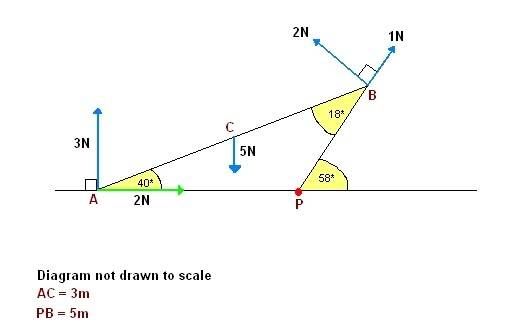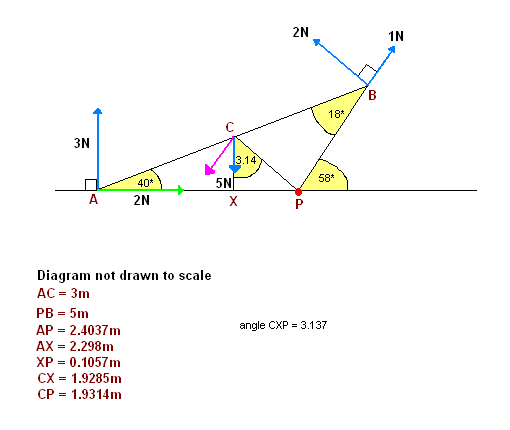# Statics of rigid bodies – calculating the moments about a point. A Lvl exam in 4 days

Hi :)
I’m self-studying for my A Level mechanics 2 exam (it’s in 4 days :S) and there’s a problem in my book that I just can’t solve. Any help would be really appreciated. :)

## Homework Statement

Find the sum of the moments about the point P of the forces shown in the diagram:## Homework Equations

Moment about a point = Force x Perpendicular distance of the force from the point

## The Attempt at a Solution

From the way the problem diagram is shown in my book, it seems as though APB is a rigid triangular frame.

Since we’re finding the sum of the moments about the point P, we won’t be considering the green 2N force and the blue 1N force because they both act along P. We won’t be considering the 5N force either because it doesn’t have any effect on the moments about P.

Moment of the blue 2N force about P
= 2N x PB
= 2N x 5m
= 10 Nm anticlockwise

Applying the sine rule:
(AP / sin 18 degrees) = (PB / sin 40 degrees)
Or, AP = sin 18 degrees x (5m / sin 40 degrees)
Hence, AP = 2.4037m

Moment of the 3N force about P
= 3N x 2.4037m
= 7.2111 Nm clockwise

= 10 Nm – 7.2111Nm
= 2.7889Nm anticlockwise
= 2.79 Nm anticlockwise

My book’s answer is 2.07 Nm anticlockwise. Could someone please tell me where I’m going wrong?

What about the force being applied at point C?

Americanforest, how does the force being applied at point C have an effect on P? The diagram is confusing me quite a bit. The fact that the triangle is a wire frame just confuses me further -- which forces act on P and which don't?. I'm not very good at mechanics, and I'm self-studying too, so some of the really self-explanatory things don't make sense to me. My book's not very useful at explaining things either :( If you could explain this problem, it'd be really helpful :)

Torque $$\vec{\tau}=\vec{r} \times \vec{F}$$ so that $$\left| \tau \right|=rFsin(\theta)$$ where theta is the angle between the force and the vector which goes from, in our case, P, to the point at which the force is applied. This torque is not zero for the force applied at point C.

Americanforest, based on the info you've given me, I've made some additions to my diagram. So will I now be taking the moment of the pink force (vertical component of the blue 5N force) about the point P?Pink force = 5N x sin 3.137 degrees

Moment
= CP x (5N x sin 3.137 degrees)
= 0.528 Nm anticlockwise

However, this just takes my answer further away from the book's answer of 2.07Nm anticlockwise.

Sum of anticlockwise moments
= 10 Nm + 0.528Nm
= 10.528 Nm

Sum of clockwise moments
= 3N x 2.4037m
= 7.2111 Nm

Overall sum of moments about P
= 3.3169 Nm
= 3.31 Nm anticlockwise

Where am I going wrong?

PhanthomJay
Homework Helper
Gold Member

Well, assuming your geometry is correct , your method now looks OK. I didn't check your geometry and trig too closely, so I can't be sure of the numbers, but it looks OK; it appears that the book answer may be wrong, based on your given values. Nevertheless, the concept is to sum moments of all forces about the given point, watching cw and ccw signs, using the fact that the moment of a force is force times perpendicular distance, or rFsin theta if you wish. You calculated the Moment of the force at C about P as 5(sin 3.14)(CP), ccw, or you could have done it as 5(XP), ccw, and achieve the same result.

Hi :)
I’m self-studying for my A Level mechanics 2 exam (it’s in 4 days :S) and there’s a problem in my book that I just can’t solve. Any help would be really appreciated. :)

## Homework Statement

Find the sum of the moments about the point P of the forces shown in the diagram:## Homework Equations

Moment about a point = Force x Perpendicular distance of the force from the point

## The Attempt at a Solution

From the way the problem diagram is shown in my book, it seems as though APB is a rigid triangular frame.

Since we’re finding the sum of the moments about the point P, we won’t be considering the green 2N force and the blue 1N force because they both act along P. We won’t be considering the 5N force either because it doesn’t have any effect on the moments about P.

Moment of the blue 2N force about P
= 2N x PB
= 2N x 5m
= 10 Nm anticlockwise

Applying the sine rule:
(AP / sin 18 degrees) = (PB / sin 40 degrees)
Or, AP = sin 18 degrees x (5m / sin 40 degrees)
Hence, AP = 2.4037m

Moment of the 3N force about P
= 3N x 2.4037m
= 7.2111 Nm clockwise

= 10 Nm – 7.2111Nm
= 2.7889Nm anticlockwise
= 2.79 Nm anticlockwise

My book’s answer is 2.07 Nm anticlockwise. Could someone please tell me where I’m going wrong?

You need to consider point C force. Calculte this one.
10+5(2.4037-3cos40-5cos58)-7.211

Inky, that gives me -9.9311, which is even further off from the book's answer :(

Jay, I hope you're right :)

Thank-you everyone for trying to help :)

PhanthomJay